# Maharashtra SSC Board (MSBSHSE) Question Paper for Class 10th Science Paper 1 2018 In PDF

## MSBSHSE 10th Standard Science Paper 1 Exam Question Papers 2018 with Solutions – Free Download

MSBSHSE (Maharashtra board) Class 10 Science Part I 2018 Question Paper with solutions are provided in the PDF format, here at BYJU’S. This solved SSC Science Paper 1 Question paper can be accessed at any time for free by just clicking on the downloadable links provided in the article. Science is a challenging subject for the students and to score well in it, they will have to master all the concepts related to the subject.

Students can easily score full marks in MSBSHSE Class 10 Science Part I exam if they have learnt all the complex concepts of the chapter well. Practice of all the previous papers of msbshse class 10 science will help the students to understand the exam pattern as well as the type of questions that are likely to be asked in the board exams. This, in turn, helps the students in solving various types of questions that appear in the annual exam of Maharashtra board and thus score well.

### MSBSHSE Class 10 Science Part I 2018 Question Paper with Solutions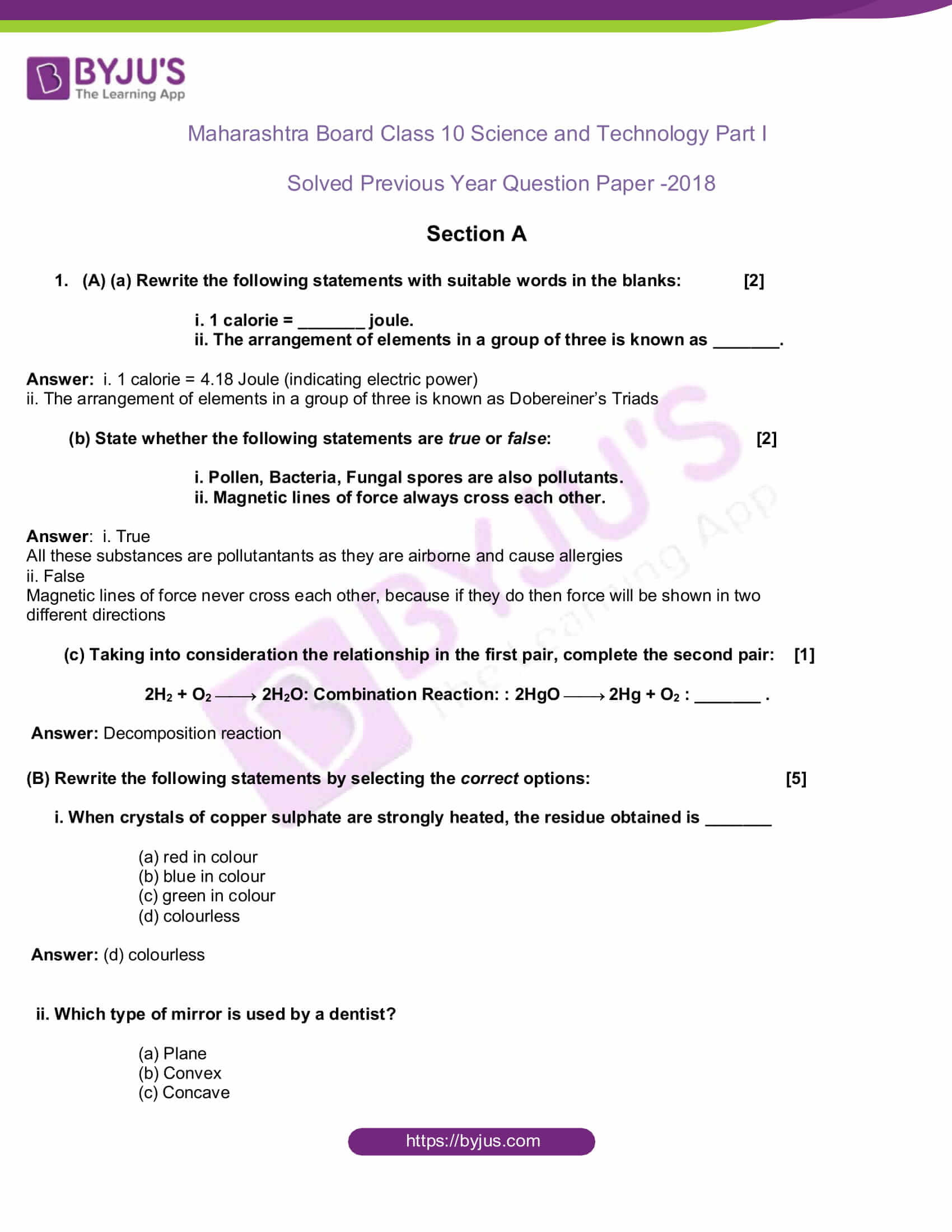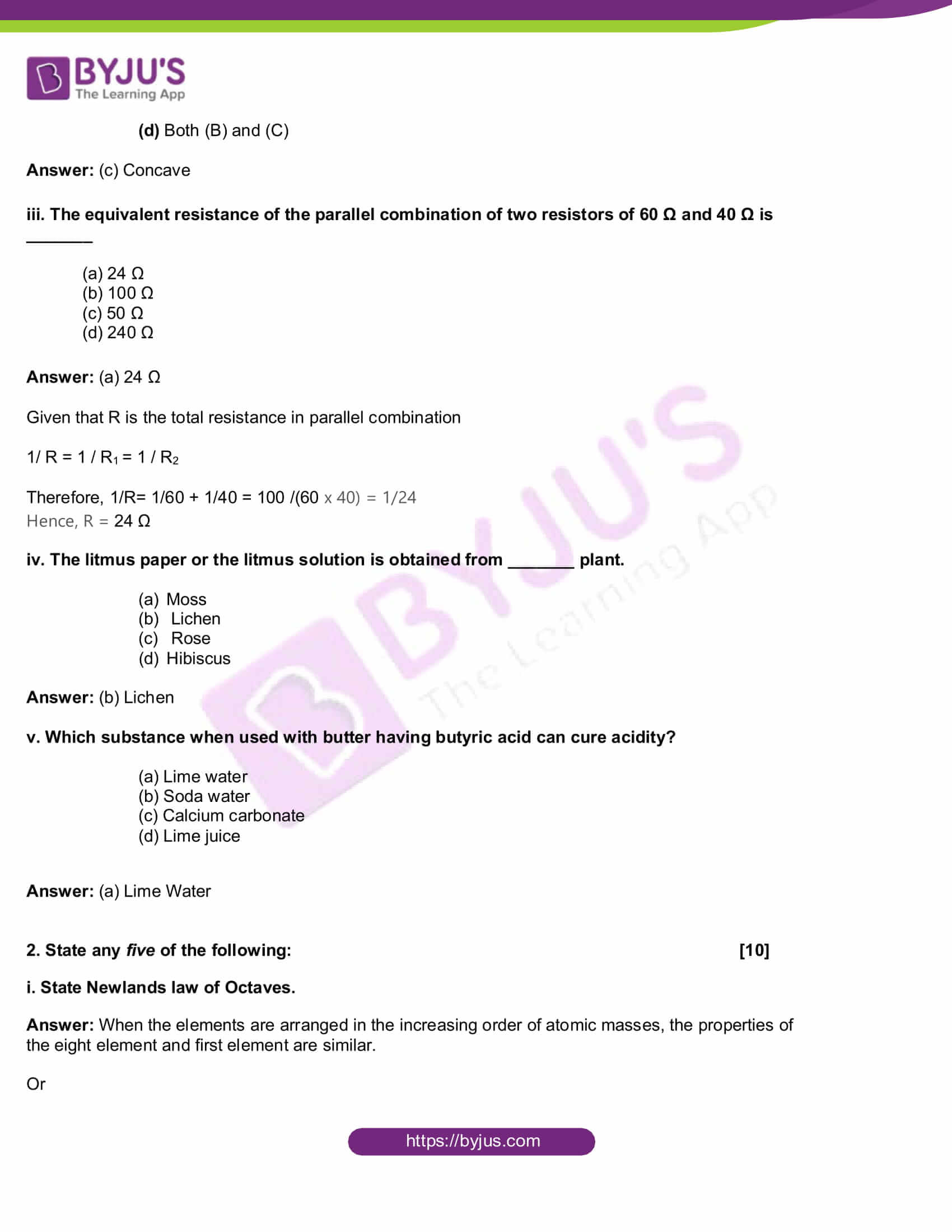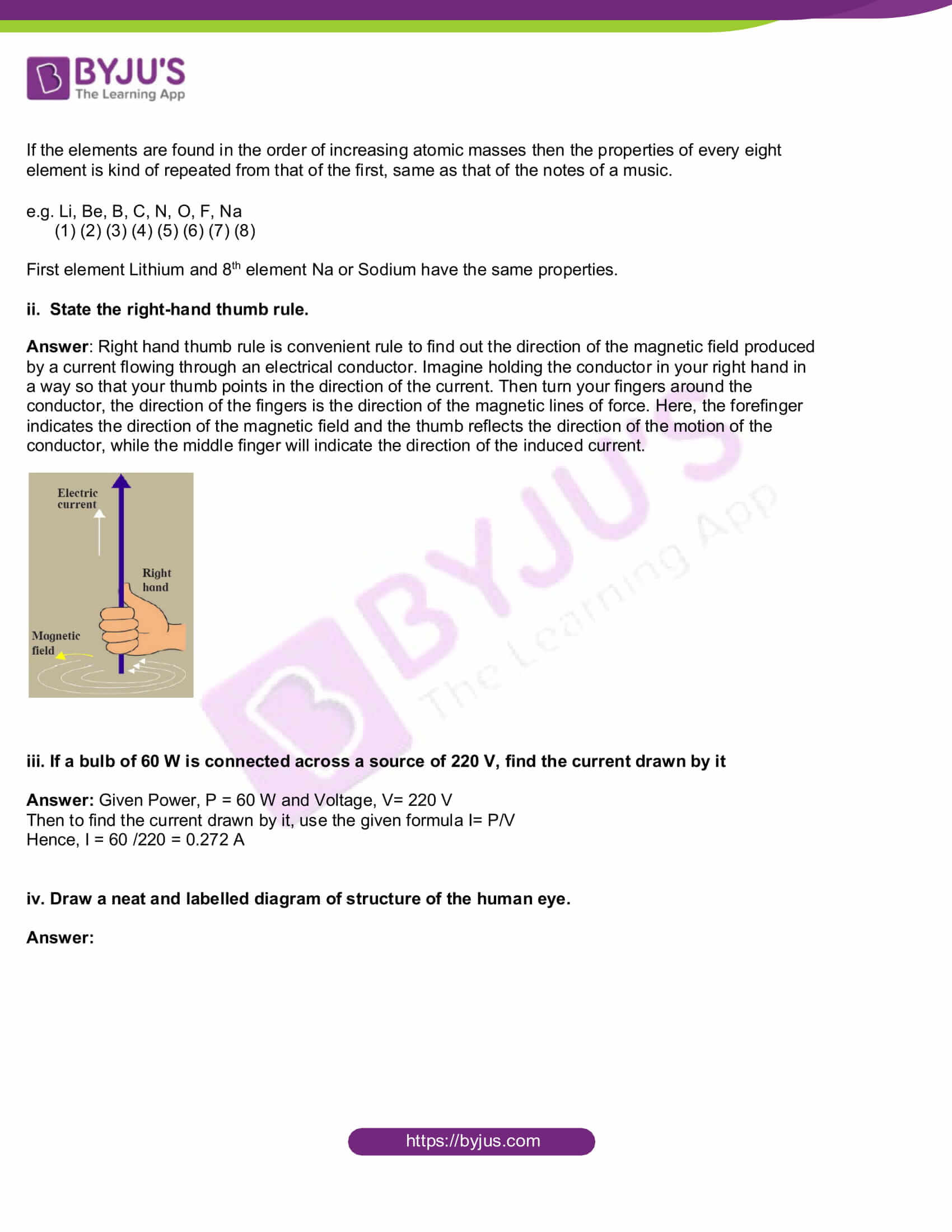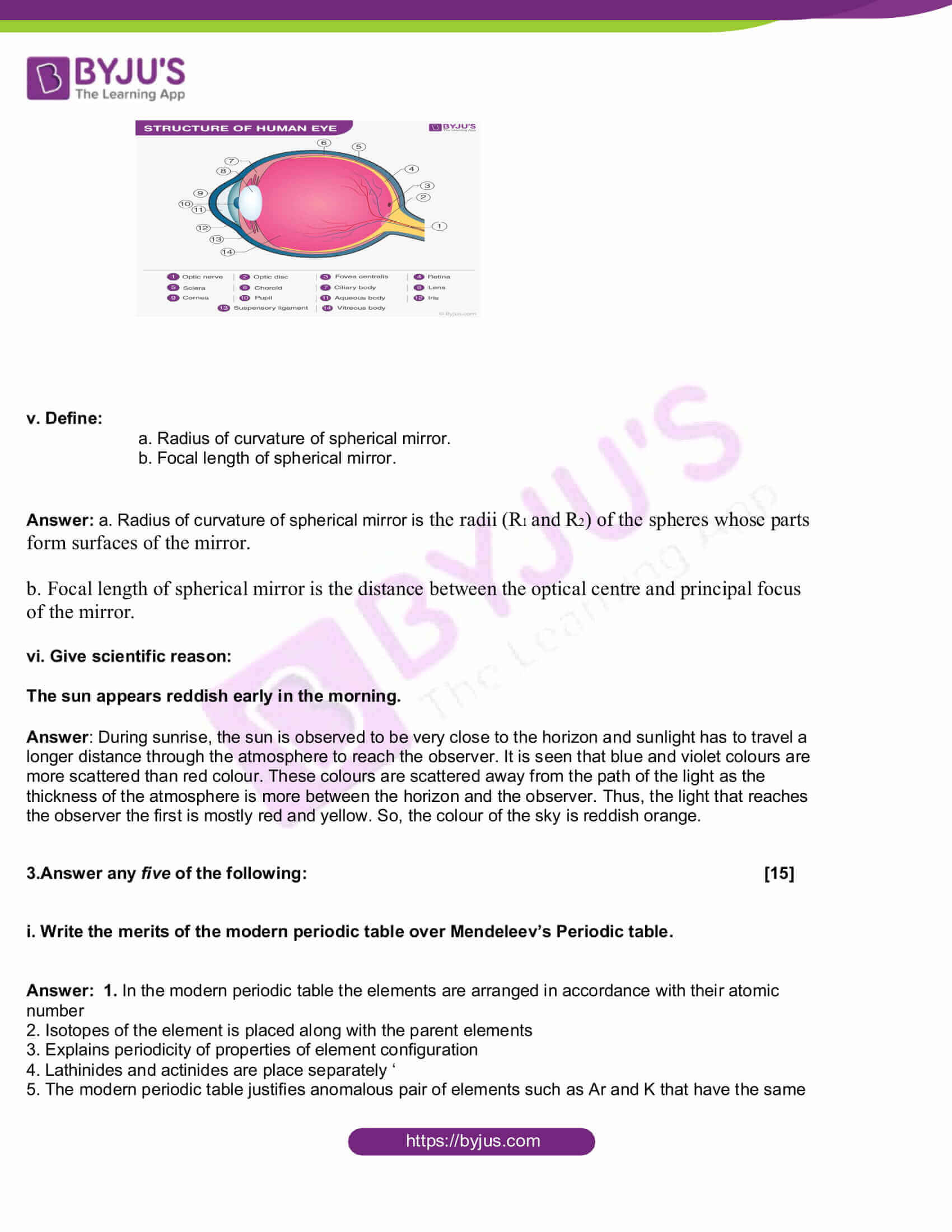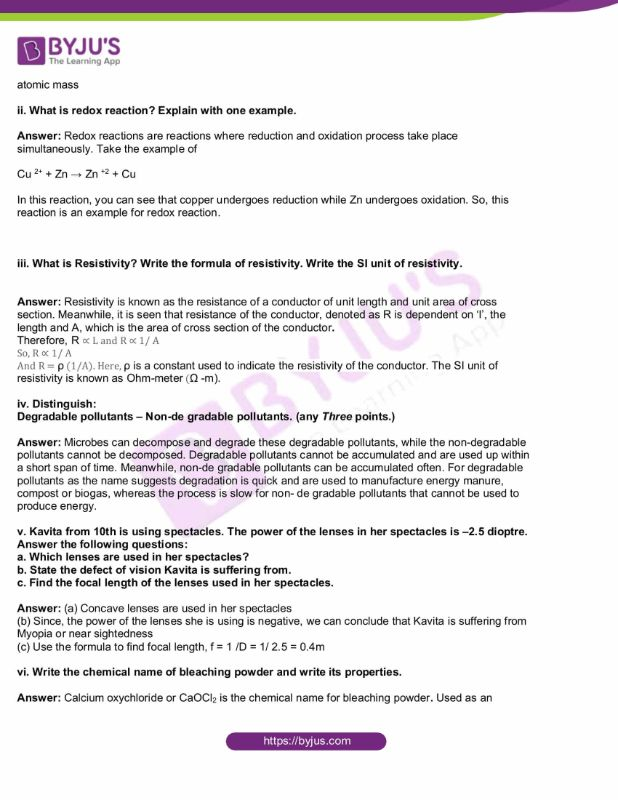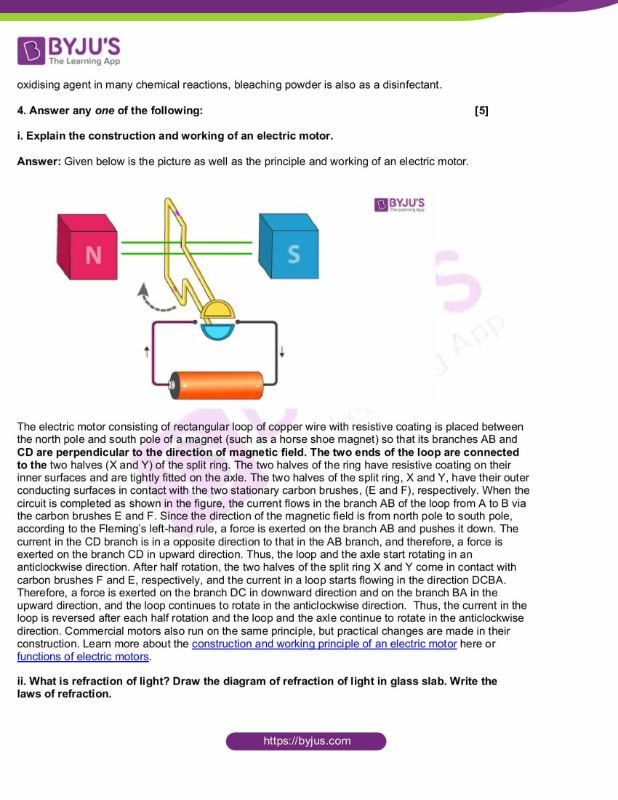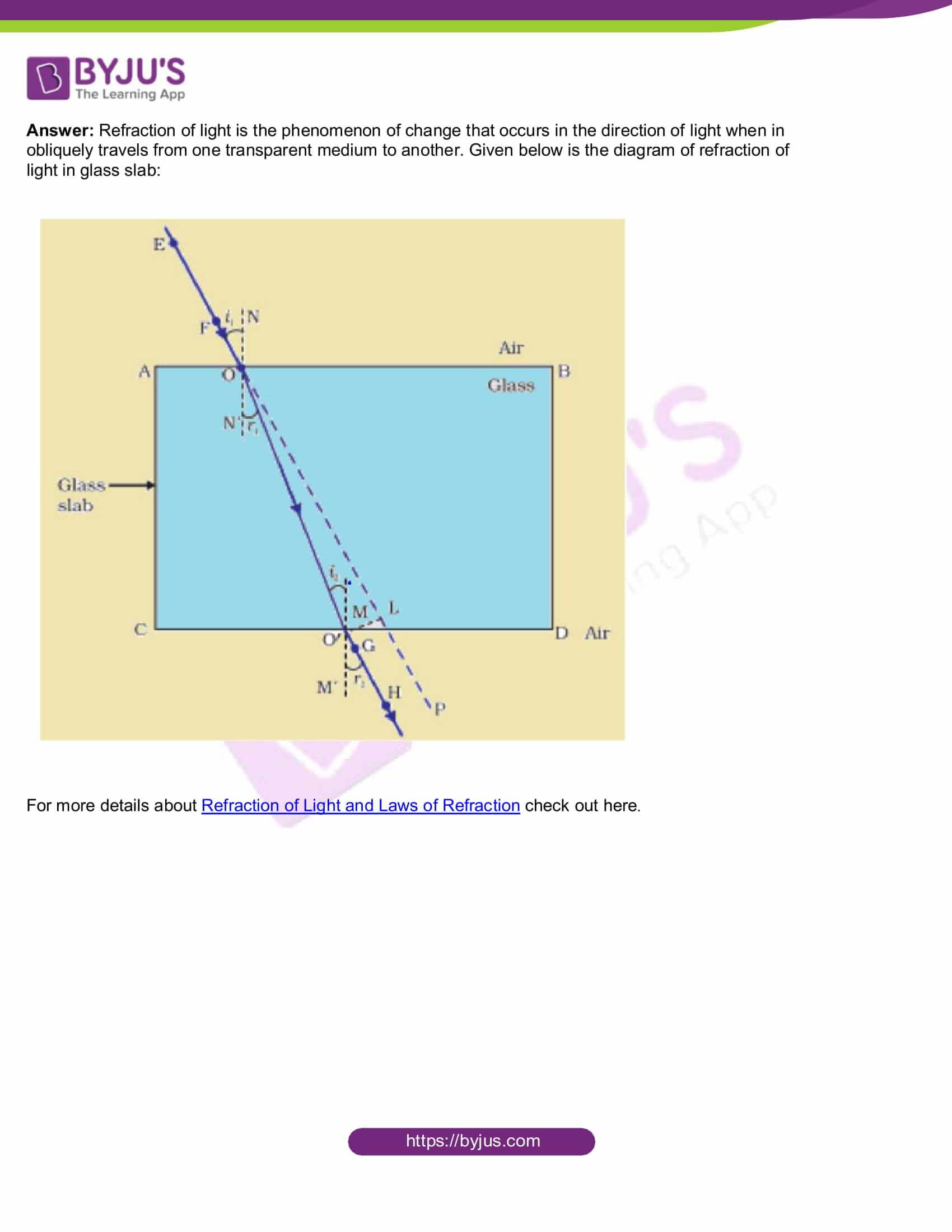Section A

1. (A) (a) Rewrite the following statements with suitable words in the blanks: 

i. 1 calorie = _______ joule.

ii. The arrangement of elements in a group of three is known as _______.

Answer: i. 1 calorie = 4.18 Joule (indicating electric power)

ii. The arrangement of elements in a group of three is known as Dobereiner’s Triads

(b) State whether the following statements are true or false: 

i. Pollen, Bacteria, Fungal spores are also pollutants.

ii. Magnetic lines of force always cross each other.

All these substances are pollutantants as they are airborne and cause allergies

ii. False

Magnetic lines of force never cross each other, because if they do then force will be shown in two different directions.

(c) Taking into consideration the relationship in the first pair, complete the second pair: 

2H2 + O2 ¾¾ 2H2O: Combination Reaction: : 2HgO ¾¾ 2Hg + O2 : _______ .

(B) Rewrite the following statements by selecting the correct options: 

i. When crystals of copper sulphate are strongly heated, the residue obtained is _______

(a) red in colour

(b) blue in colour

(c) green in colour

(d) colourless

ii. Which type of mirror is used by a dentist?

(a) Plane

(b) Convex

(c) Concave

(d) Both (B) and (C)

iii. The equivalent resistance of the parallel combination of two resistors of 60 Ω and 40 Ω is _______

(a) 24 Ω

(b) 100 Ω

(c) 50 Ω

(d) 240 Ω

Given that R is the total resistance in parallel combination

1/ R = 1 / R1 = 1 / R2

Therefore, 1/R= 1/60 + 1/40 = 100 /(60 x 40) = 1/24

Hence, R = 24 Ω

iv. The litmus paper or the litmus solution is obtained from _______ plant.

(a) Moss

(b) Lichen

(c) Rose

(d) Hibiscus

v. Which substance when used with butter having butyric acid can cure acidity?

(a) Lime water

(b) Soda water

(c) Calcium carbonate

(d) Lime juice

2. State any five of the following: 

i. State Newlands law of Octaves.

Answer: When the elements are arranged in the increasing order of atomic masses, the properties of the eight element and first element are similar.

Or

If the elements are found in the order of increasing atomic masses then the properties of every eighth element is kind of repeated from that of the first, same as that of the notes of a music.

e.g. Li, Be, B, C, N, O, F, Na

(1) (2) (3) (4) (5) (6) (7) (8)

First element Lithium and 8th element Na or Sodium have the same properties.

ii. State the right-hand thumb rule.

Answer: Right hand thumb rule is a convenient rule to find out the direction of the magnetic field produced by a current flowing through an electrical conductor. Imagine holding the conductor in your right hand in a way so that your thumb points in the direction of the current. Then turn your fingers around the conductor, the direction of the fingers is the direction of the magnetic lines of force. Here, the forefinger indicates the direction of the magnetic field and the thumb reflects the direction of the motion of the conductor, while the middle finger will indicate the direction of the induced current.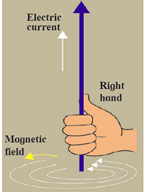iii. If a bulb of 60 W is connected across a source of 220 V, find the current drawn by it

Answer: Given Power, P = 60 W and Voltage, V= 220 V

Then to find the current drawn by it, use the given formula I= P/V

Hence, I = 60 /220 = 0.272 A

iv. Draw a neat and labelled diagram of structure of the human eye.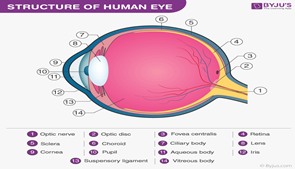v. Define:

a. Radius of curvature of spherical mirror.

b. Focal length of spherical mirror.

Answer: a. Radius of curvature of a spherical mirror is the radii (R1 and R2) of the spheres whose parts form the surfaces of the mirror.

b. Focal length of spherical mirror is the distance between the optical centre and principal focus of the mirror.

vi. Give scientific reason:

The sun appears reddish early in the morning.

Answer: During sunrise, the sun is observed to be very close to the horizon and sunlight has to travel a longer distance through the atmosphere in order to reach the observer. It is seen that blue and violet colours are more scattered than red colour. Hence, these colours are scattered away from the path of the light because the thickness of the atmosphere is more between the horizon and the observer. Thus, the light that reaches the observer first is mostly red and yellow. So, the colour of the sky is reddish orange.

3.Answer any five of the following: 

i. Write the merits of the modern periodic table over Mendeleev’s Periodic table.

Answer: 1. In the modern periodic table the elements are arranged in accordance with their atomic number

2. Isotopes of the element is placed along with the parent elements

3. Explains periodicity of properties of element configuration

4. Lathinides and actinides are placed separately

5. The modern periodic table justifies anomalous pair of elements such as Ar and K that have the same atomic mass

ii. What is a redox reaction? Explain with one example.

Answer: Redox reactions are reactions where reduction and oxidation process take place simultaneously. Take the example of

Cu 2+ + Zn → Zn +2 + Cu

In this reaction, you can see that copper undergoes reduction while Zn undergoes oxidation. So, this reaction is an example of a redox reaction.

iii. What is Resistivity? Write the formula of resistivity. Write the SI unit of resistivity.

Answer: Resistivity is known as the resistance of a conductor of unit length and unit area of cross section. Meanwhile, it is seen that resistance of the conductor, denoted as R is dependent on ‘l’, the length and A, which is the area of the cross section of the conductor.

Therefore, R ∝ L and R ∝ 1/ A

So, R ∝ 1/ A

And R = ρ (1/A). Here, ρ is a constant used to indicate the resistivity of the conductor. The SI unit of resistivity is known as Ohm-meter (Ω -m).

iv. Distinguish:

Answer: Microbes can decompose and degrade these degradable pollutants, while the non-degradable pollutants cannot be decomposed. Degradable pollutants cannot be accumulated and are used up within a short span of time. Meanwhile, non-de gradable pollutants can be accumulated often. For degradable pollutants as the name suggests degradation is quick and is used to manufacture energy manure, compost or biogas, whereas the process is slow for non- degradable pollutants that cannot be used to produce energy.

v. Kavita from 10th is using spectacles. The power of the lenses in her spectacles is –2.5 dioptre.

a. Which lenses are used in her spectacles?

b. State the defect of vision Kavita is suffering from.

c. Find the focal length of the lenses used in her spectacles.

Answer: (a) Concave lenses are used in her spectacles

(b) Since, the power of the lenses she is using is negative, we can conclude that Kavita is suffering from Myopia or nearsightedness

(c) Use the formula to find focal length, f = 1 /D = 1/ 2.5 = 0.4m

vi. Write the chemical name of bleaching powder and write its properties.

Answer: Calcium oxychloride or CaOCl2 is the chemical name for bleaching powder. Used as an oxidising agent in many chemical reactions, bleaching powder is also as a disinfectant.

4. Answer any one of the following: 

i. Explain the construction and working of an electric motor.

Answer: Given below is the picture as well as the principle and working of an electric motor.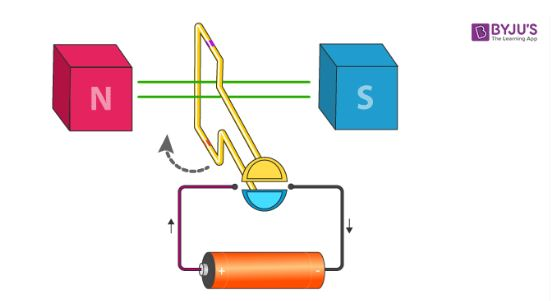The electric motor consisting of a rectangular loop of copper wire with resistive coating is placed between the north pole and south pole of a magnet (such as a horse shoe magnet) so that its branches AB and CD are perpendicular to the direction of magnetic field. The two ends of the loop are connected to the two halves (X and Y) of the split ring. The two halves of the ring have resistive coating on their inner surfaces and are tightly fitted on the axle. The two halves of the split ring, X and Y, have their outer conducting surfaces in contact with the two stationary carbon brushes, (E and F), respectively. When the circuit is completed as shown in the figure, the current flows in the branch AB of the loop from A to B via the carbon brushes E and F. Since the direction of the magnetic field is from north pole to south pole, according to the Fleming’s left-hand rule, a force is exerted on the branch AB and pushes it down. The current in the CD branch is in the opposite direction to that in the AB branch, and therefore, a force is exerted on the branch CD in upward direction. Thus, the loop and the axle start rotating in an anticlockwise direction. After half rotation, the two halves of the split ring X and Y come in contact with carbon brushes F and E, respectively, and the current in a loop starts flowing in the direction DCBA. Therefore, a force is exerted on the branch DC in downward direction and on the branch BA in the upward direction, and the loop continues to rotate in the anticlockwise direction. Thus, the current in the loop is reversed after each half rotation and the loop and the axle continue to rotate in the anticlockwise direction. Commercial motors also run on the same principle, but practical changes are made in their construction. Learn more about theconstruction and working principle of an electric motor here or functions of electric motors.

ii. What is refraction of light? Draw the diagram of refraction of light in a glass slab. Write the laws of refraction.

Answer: Refraction of light is the phenomenon of change that occurs in the direction of light when it obliquely travels from one transparent medium to another. Given below is the diagram of refraction of light in glass slab: